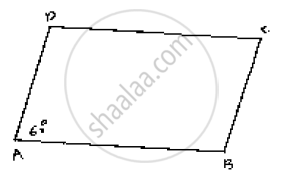# The Opposite Sides of a Quadrilateral Are Parallel. If One Angle of the Quadrilateral is 60°, Find the Other Angles. - Mathematics

The opposite sides of a quadrilateral are parallel. If one angle of the quadrilateral is 60°,
find the other angles.

#### SolutionGiven AB || CD

Since  AB || CD and AD is the transversal

∠A + ∠D = 180°     [Co-interior angles are supplementary]

60° + ∠D = 180°

∠D = 180° - 60°

∠D = 120°

Now, AD || BC and AB is the transversal

∠A + ∠B = 180°            [Co-interior angles are supplementary]

60° + ∠B = 180°

∠B = 180° - 60° = 120°

Hence ∠A = ∠C = 60°

ÐB = ÐD =120°

Concept: Pairs of Angles
Is there an error in this question or solution?

#### APPEARS IN

RD Sharma Mathematics for Class 9
Chapter 10 Lines and Angles
Exercise 10.4 | Q 12 | Page 47